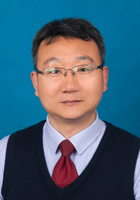Home>Science

## Numerical Analysis

Nanjing University of Aeronautics and Astronautics| Updated: September 18, 2020

About the course

This course is an important basic course for specialty for undergraduate students of non-mathematical majors. This course will emphasize the development of numerical algorithms to provide solutions to common problems formulated in science and engineering. The primary objective of the course is to develop the basic understanding of the construction of numerical algorithms, and perhaps more importantly, the applicability and limits of their appropriate use. It is to introduce the students to the extent to which numerical methods are needed for solving various scientific problems, and to train them to use these methods to find approximate solutions to the issues raised in various fields of research.

This course mainly covers the classical theory and methods of numerical analysis theory, including solution of nonlinear equations, solution of systems of linear equations, approximation of functions, numerical integration and numerical solution of ordinary differential equations.

It requires that students should master the basic discipline of numerical analysis, be able to carry out the classical numerical methods for some mathematical problems and have the basic knowledge and skills to use computers to solve mathematical problems and conduct research by both algorithm-designing and computer programming so that they are prepared with the necessary foundation for their future study and work.

About the teacherZhengsheng Wang, Professor of Mathematics

Department of Mathematics, College of Science

Nanjing University of Aeronautics and Astronautics

Email: wangzhengsheng@nuaa.edu.cn

Zhengsheng Wang received his Ph.D. degree from Nanjing University of Aeronautics and Astronautics (NUAA) in 2006. He is now a full professor in College of Science at NUAA and teaches the courses of Numerical Analysis, Matrix Theory and Computation and Applied Software for the graduate students, undergraduate students and international students. His research has been focused on Numerical Analysis, Scientific Computing, Matrix Theory and Computations with Applications. He has published more than 30 academic papers and 6 books. He is one of the most popular and outstanding teachers in NUAA.

All materials are contributed by Nanjing University of Aeronautics and Astronautics.

Cities>